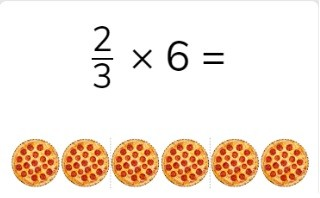Multiplying fractions with whole numbers

# Multiplying fractions with whole numbers

I can multiply a fraction with a whole number.8,000 schools use Gynzy92,000 teachers use Gynzy1,600,000 students use Gynzy

## General

Students learn to multiply a fraction with a whole number.

## Standards

CCSS.Math.Content.5.NF.B

## Introduction

Have students simplify the given fractions and convert them to mixed numbers.

## Instruction

Remind students that fractions are made of a numerator and a denominator. Show the problem 2//3 x 6. Explain that you are going to determine what 2//3 of 6 pizzas is. Begin by dividing the whole number by the denominator of the fraction (6 ÷ 3 = 2). Then multiply the quotient by the numerator of the fraction (2 x 2 = 4). That means you have 4 whole pizzas. Ask students to solve the next problems individually or in pairs with the problems with visual support of a fraction circle. Next show the problem 2//6 x 24. Explain that again, they start by dividing the whole number by the denominator (24 ÷ 6 = 4). Next they multiply the quotient by the numerator of the fraction (4 x 2 = 8). Ask students to solve the next problems individually or in pairs. Remind students how to solve fraction word problems- by finding the numbers or fractions required for the word problem, say or write the problem and then solve. Solve the first fraction word problem together, and then ask students to solve the next individually or in pairs.

To check that students can multiply a fraction with a whole number, you can ask the following question:
- Which steps do you take to multiply a fraction with a whole number?

## Quiz

Students are first given a multiplication problem with visual support for the fraction. Then they are given abstract problems, and finally fraction story problems to solve.

## Closing

Discuss with students why it is important to be able to multiply a fraction to a whole number. To close, ask students to create a fraction in the fraction circle, spin the dial and then solve the problem that they have created.

## Teaching tips

Students who have difficulty multiplying fractions by whole numbers, can first practice multiplying unit fractions with low numbers. They can also use a fraction circle or fraction bar to help visualize the process.

## Instruction materials

Optional: worksheets or otherwise with fraction circles or bars, and other fraction representations.

### The online teaching platform for interactive whiteboards and displays in schools

• Save time building lessons

• Manage the classroom more efficiently

• Increase student engagement